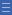## Featured resource

Home > Topdrawer > Mental computation > Activities > How many possible ways?

# How many possible ways?

How many possible ways?

In this activity, students explore the different arrays that can be formed for a specific number.

Factors are pairs of counting numbers that multiply to give another counting number.

Constraining the number of tiles and building different rectangular arrays develops the concept of factors and the commutative property of multiplication.

Pose the following situation:

I made an array with 24 tiles. What might it look like? Close your eyes and picture it. Open your eyes and make what you saw.

Note the way the students work out the different arrays. Do they recognise that by rotating the structure they generate another arrangement?

Students share the different possibilities and draw them on the board or interactive whiteboard.

• What is the same about all the arrays?
• What is different?
• What do you notice about the number of rows and number of tiles in each row?

Pose extension problems such as:

I wonder if another number such as 36 would have as many different possible arrays as 24?

Yes

Yes

Name Class SectionYear 4: Recall multiplication facts up to 10 x 10 and related division facts Infobox 3Year 3: Recall multiplication facts of two, three, five and ten and related division facts Infobox 3Source Infobox 3